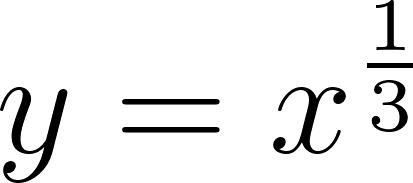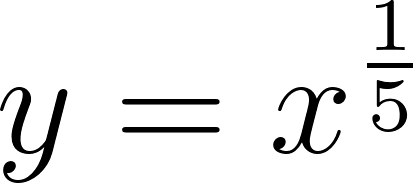×
Get Full Access to Calculus: Early Transcendentals - 1 Edition - Chapter 1.2 - Problem 54e
Get Full Access to Calculus: Early Transcendentals - 1 Edition - Chapter 1.2 - Problem 54e

×

# Answer: Roots and powers. M ake a rough sketch of theISBN: 9780321570567 2

## Solution for problem 54E Chapter 1.2

Calculus: Early Transcendentals | 1st Edition

• Textbook Solutions
• 2901 Step-by-step solutions solved by professors and subject experts
• Get 24/7 help from StudySoup virtual teaching assistantsCalculus: Early Transcendentals | 1st Edition

4 5 1 366 Reviews
13
4
Problem 54E

Roots and powers: Make a rough sketch of the given pairs of functions. Be sure to draw the graphs accurately relative to each other.andStep-by-Step Solution:

Step 1 of 2

Given functions,andWe need to draw the graph of given pairs of functions.

Step 2 of 2

##### ISBN: 9780321570567

Calculus: Early Transcendentals was written by and is associated to the ISBN: 9780321570567. The full step-by-step solution to problem: 54E from chapter: 1.2 was answered by , our top Calculus solution expert on 03/03/17, 03:45PM. This full solution covers the following key subjects: accurately, ake, draw, functions, given. This expansive textbook survival guide covers 112 chapters, and 5248 solutions. The answer to “?Roots and powers: Make a rough sketch of the given pairs of functions. Be sure to draw the graphs accurately relative to each other. and” is broken down into a number of easy to follow steps, and 25 words. This textbook survival guide was created for the textbook: Calculus: Early Transcendentals, edition: 1. Since the solution to 54E from 1.2 chapter was answered, more than 410 students have viewed the full step-by-step answer.

Unlock Textbook Solution